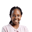Related Tags

machine learningJoy Kareko

### Overview

Machine learning is the science of teaching computers to identify underlying patterns in data. Machine learning uses an appropriate algorithm to obtain a model that generalizes well on the dataset and gives accurate predictions on unseen data.

Loss functions (also known as cost/error functions) help us evaluate how well a model performs when it comes to predictions. This is why model optimization is an important step in machine learning. Optimization helps us to systematically and iteratively minimize the loss and obtain a model that can generalize well on the data and therefore predict accurately.

Two of the most common optimization algorithms used to minimize the loss function in a machine learning or deep learning model are:

### How the gradient descent works

Let’s assume we are working on a machine learning model and have obtained the various parameters of our features.

A good model is able to give accurate predictions, so if a model performs poorly, it means the error is high. In this case, we’ll need to minimize it. But how exactly do we minimize the error? This is where gradient descent comes in.

Gradient descent is a technique used to obtain the local minimum of any function in an iterative process.

The Gradient descent finds the slope/gradient to the loss function at a certain random point, then descends down that slope in small continuous steps until the gradient reaches its minimum, that is, zero. This process updates the parameters and calculates a new cost function. These steps are determined by a hyperparameter known as a learning rate.

The learning rate is an important hyperparameter in this case and has to be tuned to achieve the best results.

If the learning rate is too high, the gradient will ‘jump’ across the particular curve, missing out on the minima.

If it is too small, the iterative process will take a long time, making it computationally expensive.

Image showing Gradient Descent on a Cost Function

### How the stochastic gradient descent works

Stochastic gradient descent is a variant of gradient descent. In gradient descent, all data points are used to obtain new parameters of a model at each step, and then a new cost function is calculated. If we have a large sample, this becomes computationally expensive.

One solution to this is by using stochastic gradient descent.

The word stochastic means random, and here is how it works. Instead of using the whole dataset to obtain new weights/parameters and then obtaining a new cost function, the stochastic gradient descent chooses one random point at each iteration to calculate new weights/parameters as well as a cost function and then repeats the process until the cost function reaches its minima. This makes it faster.

However, the downside is that the use of random data points to obtain new weights/parameters generates random updates and oscillations to the cost function making it noisy. While this might help reach the local minima quickly, the opposite is also true. Frequent updates can also make the gradients jump off and prevent them from reaching the minima quickly.

RELATED TAGS

machine learning

CONTRIBUTORJoy Kareko